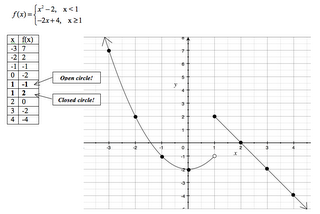## Thursday, October 12, 2006

### Next Lesson: More Piecewise FunctionsThis lesson will start out with students warming up on a piecewise function with only linear parts, as in the homework. The graph is followed by a series of evaluation problems. The first can be done by looking at the graph - the rest require use of the equations. This will be explained later in the class; for now, the idea is to see if students can figure out how to do it, either alone or by working together.

After reviewing this, we will add to the notes from the previous lesson. I'll show the students how to evaluate a piecewise function algebraically by determining which condition is made true by the x-value, and then substituting the x-value into the corresponding equation. We can use this idea to go back to the Do Now problems if they didn't figure them out. I will set up an x-y table to record the values we test during this portion of the lesson - next, we'll use tables to graph more complicated functions.

At this point, I'll hand out a reading to the students that shows how to use a table to help graph a piecewise function that has non-linear pieces (like absolute values, quadratics, etc.). My idea is that they will write the x-values that are the endpoints of the conditions twice in the table, and evaluate them with each of the equation parts. They will indicate on the table if the y-value is a closed or open circle. Students will then have time to practice this independently.

Homework is a set of problems that will help them review for the second quiz in the next class.

#### 1 comment:

robert said...

I am writing a paper on the exponential rate of change (the old Alvin Toffler Future Shock idea) and I want to refer to the portion of the exponential curve where it rapidly starts approaching the vertical. I know that that part of the curve has a special name, but I can't think of it. Can you help me?